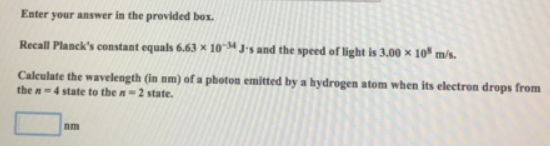# Problem: Enter your answer in the provided box.Recall Planck's constant equals 6.63 x 10-34 J • s and the speed of light is 3.00 x 108 m/s. Calculate the wavelength (in am) of a photon emitted by a hydrogen atom when its electron drops from the n = 4 state to the n = 2 state.

🤓 Based on our data, we think this question is relevant for Professor MacFarland's class at CSU.

###### FREE Expert Solution###### Problem Details

Recall Planck's constant equals 6.63 x 10-34 J • s and the speed of light is 3.00 x 108 m/s.

Calculate the wavelength (in am) of a photon emitted by a hydrogen atom when its electron drops from the n = 4 state to the n = 2 state.